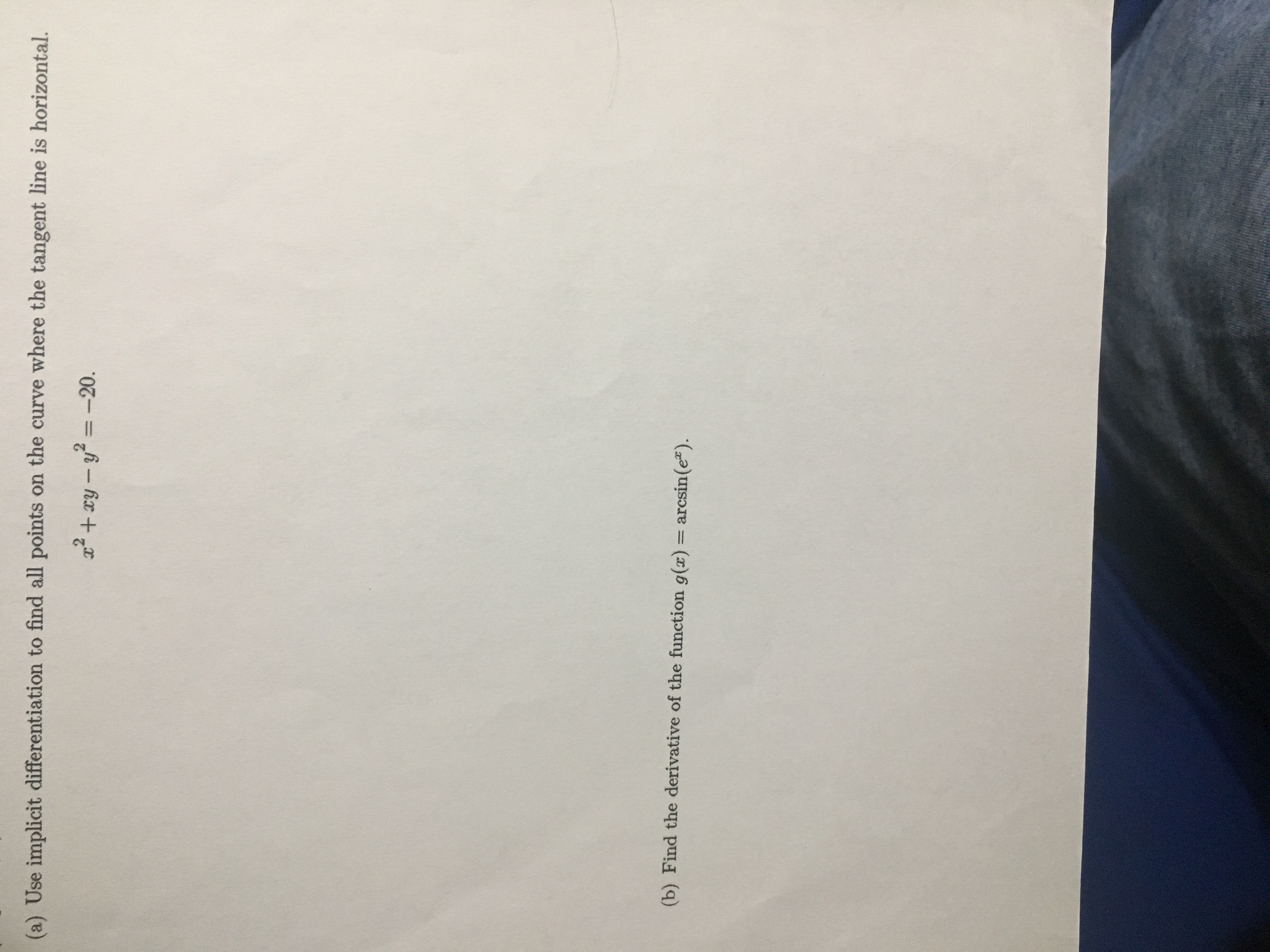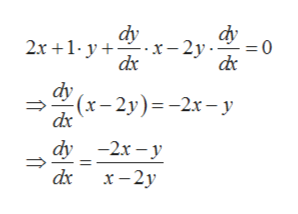# (a) Use implicit differentiation to find all points on the curve where the tangent line is horizontal.2+xy-2-20.(b) Find the derivative of the function g(x) = arcsin (e)

Question
60 viewshelp_outlineImage Transcriptionclose(a) Use implicit differentiation to find all points on the curve where the tangent line is horizontal. 2+xy-2-20. (b) Find the derivative of the function g(x) = arcsin (e) fullscreen
check_circle

star
star
star
star
star
1 Rating
Step 1

a). Given,

Step 2

Tangent line is horizontal if the slope of the tangent line is zero and slope of the tangent is the derivative of the given curve.

So differentiating the given function with respect to x, we gethelp_outlineImage Transcriptionclosedy dy х- 2у. 2х +1-у+— 0 dy (х- 2у)--2х-у dx dy -2х — у х — 2у Tm Tm fullscreen
Step 3

Now the slope of the tangent ...

### Want to see the full answer?

See Solution

#### Want to see this answer and more?

Solutions are written by subject experts who are available 24/7. Questions are typically answered within 1 hour.*

See Solution
*Response times may vary by subject and question.
Tagged in

### Derivative